# Discrete Fourier Transform (DFT)

## Arguments for the discrete implementation of the Fourier transform

The  $\text{Fourier transform}$  according to the previous description in chapter  Aperiodic Signals – Pulses has an infinitely high selectivity due to the unlimited extension of the integration interval and is therefore an ideal theoretical tool of spectral analysis.

If the spectral components  $X(f)$  of a time function  $x(t)$  are to be determined numerically, the general transformation equations are

\begin{align*}X(f) & = \int_{-\infty }^{+\infty}x(t) \cdot {\rm e}^{-{\rm j} \hspace{0.05cm}\cdot \hspace{0.05cm} 2 \pi f t}\hspace{0.1cm} {\rm d}t\hspace{0.5cm} \Rightarrow\hspace{0.5cm} \text{Transform}\hspace{0.7cm} \Rightarrow\hspace{0.5cm} \text{first Fourier integral} \hspace{0.05cm},\\ x(t) & = \int_{-\infty }^{+\infty}\hspace{-0.15cm}X(f) \cdot {\rm e}^{\hspace{0.05cm}+{\rm j} \hspace{0.05cm}\cdot \hspace{0.05cm} 2 \pi f t}\hspace{0.1cm} {\rm d}f\hspace{0.35cm} \Rightarrow\hspace{0.5cm} \text{Inverse Transform}\hspace{0.4cm} \Rightarrow\hspace{0.5cm} \text{second Fourier integral} \hspace{0.05cm}\end{align*}

unsuitable for two reasons:

• The equations apply exclusively to continuous-time signals.  With digital computers or signal processors, however, one can only process discrete-time signals.
• For a numerical evaluation of the two Fourier integrals it is necessary to limit the respective integration interval to a finite value.

$\text{This leads to the following consequence:}$

A  $\text{continuous signal}$  must undergo two processes before the numerical determination of its spectral properties, viz.

• that of  $\text{sampling}$  for discretisation, and
• that of  $\text{windowing}$  to limit the integration interval.

In the following, starting from an aperiodic time function  $x(t)$  and the corresponding Fourier spectrum  $X(f)$  a time- and frequency-discrete description suitable for computer processing is developed step by step.

## Time discretisation – Periodification in the frequency domain

The following graphs show uniformly the time domain on the left and the frequency domain on the right.  Without limiting generality,  $x(t)$  and  $X(f)$  are each real and Gaussian.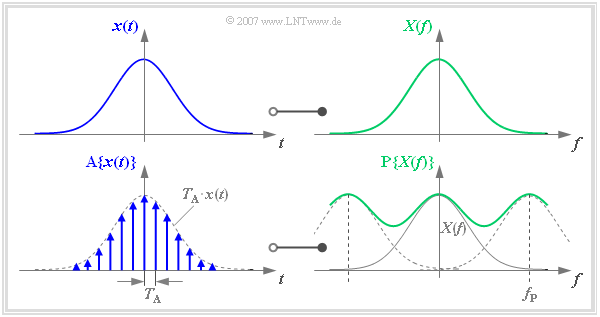Time discretisation   ⇒   Periodification in the frequency domain

According to the chapter  Discrete-Time Signal Representation  one can describe the sampling of the time signal  $x(t)$  by multiplying it by a Dirac delta train   ⇒   Dirac comb  in the time domain)

$$p_{\delta}(t) = \sum_{\nu = - \infty }^{+\infty} T_{\rm A} \cdot \delta (t- \nu \cdot T_{\rm A} )\hspace{0.05cm}.$$

The result is the time signal sampled at a distance  $T_{\rm A}$

$${\rm A}\{x(t)\} = \sum_{\nu = - \infty }^{+\infty} T_{\rm A} \cdot x(\nu \cdot T_{\rm A})\cdot \delta (t- \nu \cdot T_{\rm A} )\hspace{0.05cm}.$$

We transform this sampled signal  $\text{A}\{ x(t)\}$  into the frequency domain:

• The multiplication of the Dirac comb  $p_{\delta}(t)$  with  $x(t)$  corresponds in the frequency domain to the convolution of  $P_{\delta}(f)$  with  $X(f)$.
• The result is the periodified spectrum  $\text{P}\{ X(f)\}$, where  $f_{\rm P}$  indicates the frequency period of the function  $\text{P}\{ X(f)\}$ :
$${\rm A}\{x(t)\} \hspace{0.2cm}\circ\!\!-\!\!\!-\!\!\!-\!\!\bullet\, \hspace{0.2cm} {\rm P}\{X(f)\} = \sum_{\mu = - \infty }^{+\infty} X (f- \mu \cdot f_{\rm P} ).$$

This relation was already derived in the chapter  Discrete-Time Signal Representation  but with slightly different nomenclature:

• We now denote the sampled signal by  $\text{A}\{ x(t)\}$  instead of  $x_{\rm A}(t)$.
• The  $\text{frequency period}$  is now denoted by  $f_{\rm P} = 1/T_{\rm A}$  instead of  $f_{\rm A} = 1/T_{\rm A}$ .

These nomenclature changes are justified on the following pages.

The graph above shows the functional relationship described here. It should be noted:

• The frequency period  $f_{\rm P}$  has been deliberately chosen to be small here so that the overlap of the spectra to be summed can be clearly seen.
• In practice  $f_{\rm P}$  should be at least twice as large as the largest frequency contained in the signal  $x(t)$  due to the sampling theorem.
• If this is not fulfilled, then  $\text{Aliasing}$  must be expected - see chapter  Possible Errors when using DFT.

## Frequency discretisation – Periodification in the time domain

The discretisation of  $X(f)$  can also be described by a multiplication with a Dirac comb in the frequency domain.  The result is the sampled spectrum in the distance $f_{\rm A}$:

$${\rm A}\{X(f)\} = X(f) \cdot \sum_{\mu = - \infty }^{+\infty} f_{\rm A} \cdot \delta (f- \mu \cdot f_{\rm A } ) = \sum_{\mu = - \infty }^{+\infty} f_{\rm A} \cdot X(\mu \cdot f_{\rm A } ) \cdot\delta (f- \mu \cdot f_{\rm A } )\hspace{0.05cm}.$$

If one transforms the Dirac comb $($with impulse weights  $f_{\rm A})$  used here into the time domain, one obtains with  $T_{\rm P} = 1/f_{\rm A}$:

$$\sum_{\mu = - \infty }^{+\infty} f_{\rm A} \cdot \delta (f- \mu \cdot f_{\rm A } ) \hspace{0.2cm}\bullet\!\!-\!\!\!-\!\!\!-\!\!\circ\, \hspace{0.2cm} \sum_{\nu = - \infty }^{+\infty} \delta (t- \nu \cdot T_{\rm P } ) \hspace{0.05cm}.$$

The multiplication with  $X(f)$  corresponds in the time domain to the convolution with  $x(t)$.  One obtains the signal  $\text{P}\{ x(t)\}$  periodified in the distance  $T_{\rm P}$:

$${\rm A}\{X(f)\} \hspace{0.2cm}\bullet\!\!-\!\!\!-\!\!\!-\!\!\circ\, \hspace{0.2cm} {\rm P}\{x(t)\} = x(t) \star \sum_{\nu = - \infty }^{+\infty} \delta (t- \nu \cdot T_{\rm P } )= \sum_{\nu = - \infty }^{+\infty} x (t- \nu \cdot T_{\rm P } ) \hspace{0.05cm}.$$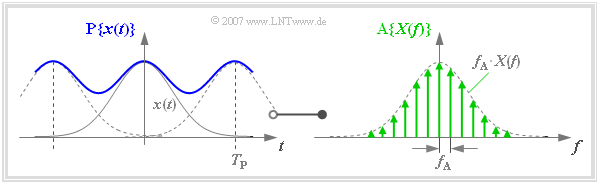Frequency discretisation – Periodisation in the time domain

$\text{Example 1:}$  This correlation is illustrated in the graph:

• Due to the coarse frequency rastering, this example results in a relatively small value for the time period  $T_{\rm P}$ .

• Therefore due to overlaps, the (blue) periodified time signal  $\text{P}\{ x(t)\}$  differs significantly from  $x(t)$.

• If one wants to achieve  $\text{P}\{ x(t)\} \approx x(t)$  then  $T_{\rm P}$  must be chosen much larger than in this example.

## Finite signal representation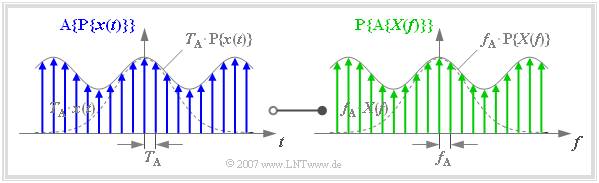Finite signals of the Discrete Fourier Transform (DFT)

One arrives at the so-called  "Finite Signal Representation" , if both

• the time function  $x(t)$
• and the spectral function  $X(f)$

are specified exclusively by their sample values.

The graph is to be interpreted as follows:

• In the left graph, the function  $\text{A}\{ \text{P}\{ x(t)\}\}$ is drawn in blue.  This results from sampling the periodified time function  $\text{P}\{ x(t)\}$  with equidistant Dirac deltas at a distance  $T_{\rm A} = 1/f_{\rm P}$.
• In the right graph, the function$\text{P}\{ \text{A}\{ X(f)\}\}$ is drawn in green.  This results from the periodification  $($with  $f_{\rm P})$  of the sampled spectral function  $\{ \text{A}\{ X(f)\}\}$.
• There is a Fourier correspondence between the blue finite signal (in the left sketch) and the green finite signal (in the right sketch), as follows:
$${\rm A}\{{\rm P}\{x(t)\}\} \hspace{0.2cm}\circ\!\!-\!\!\!-\!\!\!-\!\!\bullet\, \hspace{0.2cm} {\rm P}\{{\rm A}\{X(f)\}\} \hspace{0.05cm}.$$
• The Dirac lines of the periodic continuation  $\text{P}\{ \text{A}\{ X(f)\}\}$  of the sampled spectral function, however, only fall into the same frequency grid as those of  $\text{A}\{ X(f)\}$ if the frequency period  $f_{\rm P}$  is an integer multiple  $(N)$  of the frequency sampling interval  $f_{\rm A}$ .
• Therefore, when using the finite signal representation, the following condition must always be fulfilled, where in practice the natural number  $N$  is usually a power of two  (the above graph is based on the value  $N = 8$ ):
$$f_{\rm P} = N \cdot f_{\rm A} \hspace{0.5cm} \Rightarrow\hspace{0.5cm} {1}/{T_{\rm A}}= N \cdot f_{\rm A} \hspace{0.5cm} \Rightarrow\hspace{0.5cm} N \cdot f_{\rm A}\cdot T_{\rm A} = 1\hspace{0.05cm}.$$
• If the condition  $N \cdot f_{\rm A} \cdot T_{\rm A} = 1$  is fulfilled then the order of periodization and sampling is interchangeable.  Thus:
$${\rm A}\{{\rm P}\{x(t)\}\} = {\rm P}\{{\rm A}\{x(t)\}\}\hspace{0.2cm}\circ\!\!-\!\!\!-\!\!\!-\!\!\bullet\, \hspace{0.2cm} {\rm P}\{{\rm A}\{X(f)\}\} = {\rm A}\{{\rm P}\{X(f)\}\}\hspace{0.05cm}.$$

$\text{Conclusion:}$

• The time function  $\text{P}\{ \text{A}\{ x(t)\}\}$  has the period  $T_{\rm P} = N \cdot T_{\rm A}$.
• The period in the frequency domain is  $f_{\rm P} = N \cdot f_{\rm A}$.
• For the description of the discretised time and frequency response in each case  $N$  $\text{complex numerical values}$  in the form of impulse weights are thus sufficient.

$\text{Example 2:}$  A time-limited (pulse-like) signal  $x(t)$  is present in sampled form, where the distance between two samples is  $T_{\rm A} = 1\, {\rm µ s}$:

• After a discrete Fourier transform with  $N = 512$  the spectrum  $X(f)$  s available in form of frequency-samples at spacing  $f_{\rm A} = (N \cdot T_{\rm A})^{-1} \approx 1.953\,\text{kHz}$.
• Increasing the DFT parameter to  $N= 2048$ results in a (four times) finer frequency grid with  $f_{\rm A} \approx 488\,\text{Hz}$.

## From the continuous to the discrete Fourier transform

From the conventional first Fourier integral

$$X(f) =\int_{-\infty }^{+\infty}x(t) \cdot {\rm e}^{-{\rm j} \hspace{0.05cm}\cdot \hspace{0.05cm} 2 \pi \hspace{0.05cm}\cdot \hspace{0.05cm} f \hspace{0.05cm}\cdot \hspace{0.05cm}t}\hspace{0.1cm} {\rm d}t$$

arises from discretisation  $(\text{d}t \to T_{\rm A}$,  $t \to \nu \cdot T_{\rm A}$,  $f \to \mu \cdot f_{\rm A}$,  $T_{\rm A} \cdot f_{\rm A} = 1/N)$  the sampled and periodised spectral function

$${\rm P}\{X(\mu \cdot f_{\rm A})\} = T_{\rm A} \cdot \sum_{\nu = 0 }^{N-1} {\rm P}\{x(\nu \cdot T_{\rm A})\}\cdot {\rm e}^{-{\rm j} \hspace{0.05cm}\cdot \hspace{0.05cm} 2 \pi \hspace{0.05cm} \cdot \hspace{0.05cm}\nu \hspace{0.05cm} \cdot \hspace{0.05cm}\mu /N} \hspace{0.05cm}.$$

It is taken into account that due to the discretisation, the periodised functions are to be used in each case.

For reasons of simplified notation, we now make the following substitutions:

• The  $N$  $\text{time-domain coefficients}$  are with the variable  $\nu = 0$, ... , $N - 1$:
$$d(\nu) =. {\rm P}\left\{x(t)\right\}{\big|}_{t \hspace{0.05cm}= \hspace{0.05cm}\nu \hspace{0.05cm}\cdot \hspace{0.05cm}T_{\rm A}}\hspace{0.05cm}.$$
• Let  $N$  $\text{frequency domain coefficients}$  be associated with the variable  $\mu = 0,$ ... , $N$ – 1:
$$D(\mu) = f_{\rm A} \cdot {\rm P}\left\{X(f)\right\}{\big|}_{f \hspace{0.05cm}= \hspace{0.05cm}\mu \hspace{0.05cm}\cdot \hspace{0.05cm}f_{\rm A}}\hspace{0.05cm}.$$
• Abbreviation is written for the from  $N$  dependent  $\text{complex rotation factor}$ :
$$w = {\rm e}^{-{\rm j} \hspace{0.05cm}\cdot \hspace{0.05cm} 2 \pi /N} = \cos \left( {2 \pi}/{N}\right)-{\rm j} \cdot \sin \left( {2 \pi}/{N}\right) \hspace{0.05cm}.$$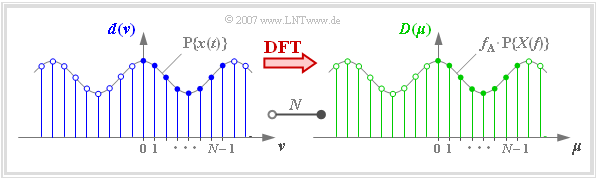On defining the  "Discrete Fourier Transform"  $\rm (DFT)$  with  $N=8$

$\text{Definition:}$  The term  $\text{Discrete Fourier Transform (DFT)}$  means the calculation of the  $N$  spectral coefficients  $D(\mu)$  from the  $N$  signal coefficients  $d(\nu)$:

$$D(\mu) = \frac{1}{N} \cdot \sum_{\nu = 0 }^{N-1} d(\nu)\cdot {w}^{\hspace{0.05cm}\nu \hspace{0.07cm} \cdot \hspace{0.05cm}\mu} \hspace{0.05cm}.$$

In the diagram you can see

• the  $N = 8$  signal coefficients  $d(\nu)$  by the blue filling,
• the  $N = 8$  spectral coefficients  $D(\mu)$  at the green filling.

## Inverse discrete Fourier transform

The Inverse Discrete Fourier Transform (IDFT) describes the  second Fourier integral

\begin{align*}x(t) & = \int_{-\infty }^{+\infty}X(f) \cdot {\rm e}^{\hspace{0.05cm}{\rm j} \hspace{0.05cm}\cdot \hspace{0.05cm} 2 \pi \hspace{0.05cm}\cdot \hspace{0.05cm} f \hspace{0.05cm}\cdot \hspace{0.05cm} t}\hspace{0.1cm} {\rm d}f\end{align*}

in discretized form:

$$d(\nu) = {\rm P}\left\{x(t)\right\}{\big|}_{t \hspace{0.05cm}= \hspace{0.05cm}\nu \hspace{0.05cm}\cdot \hspace{0.05cm}T_{\rm A}}\hspace{0.01cm}.$$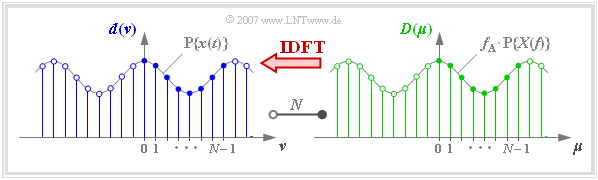On defining the  "Inverse Discrete Fourier Transform"  $\rm (IDFT)$  with  $N=8$

$\text{Definition:}$

The term  $\text{Inverse Discrete Fourier Transform (IDFT)}$  means the calculation of the signal coefficients  $d(\nu)$  from the spectral coefficients  $D(\mu)$:

$$d(\nu) = \sum_{\mu = 0 }^{N-1} D(\mu) \cdot {w}^{-\nu \hspace{0.07cm} \cdot \hspace{0.05cm}\mu} \hspace{0.05cm}.$$

With the indices  $\nu = 0, \hspace{0.05cm}\text{...} \hspace{0.05cm}, N-1,$    $\mu = 0, \hspace{0.05cm}\text{...} \hspace{0.05cm}, N-1$  holds again:

$$d(\nu) = {\rm P}\left\{x(t)\right\}{\big \vert}_{t \hspace{0.05cm}= \hspace{0.05cm}\nu \hspace{0.05cm}\cdot \hspace{0.05cm}T_{\rm A} }\hspace{0.01cm},$$
$$D(\mu) = f_{\rm A} \cdot {\rm P}\left\{X(f)\right\}{\big \vert}_{f \hspace{0.05cm}= \hspace{0.05cm}\mu \hspace{0.05cm}\cdot \hspace{0.05cm}f_{\rm A} } \hspace{0.01cm},$$
$$w = {\rm e}^{- {\rm j} \hspace{0.05cm}\cdot \hspace{0.05cm} 2 \pi /N} \hspace{0.01cm}.$$

A comparison between the  DFT  and IDFT shows that exactly the same algorithm can be used.  The only differences between IDFT and DFT are:

• The exponent of the rotation factor is to be applied with different sign.
• In the IDFT, the division by  $N$ is omitted.

## Interpretation of DFT and IDFT

The graph shows the discrete coefficients in the time and frequency domain together with the periodified continuous-time functions.

When using DFT or IDFT, please note:

1. According to the above definitions, the DFT coefficients  $d(ν)$  and  $D(\mu)$  always have the unit of the time function.
2. Dividing  $D(\mu)$  by  $f_{\rm A}$, one obtains the spectral value  $X(\mu \cdot f_{\rm A})$.
3. The spectral coefficients  $D(\mu)$  must always be complex in order to be able to consider odd time functions.
4. One also uses complex time coefficients  $d(\nu)$   ⇒   DFT and IDFT are also applicable to bandpass signals.
5. The basic interval for  $\nu$  and  $\mu$  is usually defined as the range from  $0$  to  $N - 1$  (filled circles in the graph).

With the complex-valued number sequences  $\langle \hspace{0.1cm}d(\nu)\hspace{0.1cm}\rangle = \langle \hspace{0.1cm}d(0), \hspace{0.05cm}\text{...} \hspace{0.05cm} , d(N-1) \hspace{0.1cm}\rangle$   and   $\langle \hspace{0.1cm}D(\mu)\hspace{0.1cm}\rangle = \langle \hspace{0.1cm}D(0), \hspace{0.05cm}\text{...} \hspace{0.05cm} , D(N-1) \hspace{0.1cm}\rangle$  DFT and IDFT are symbolised similarly to the conventional Fourier transform:

$$\langle \hspace{0.1cm} D(\mu)\hspace{0.1cm}\rangle \hspace{0.2cm}\bullet\!\!-\!\!\!-(N)\!-\!\!\!-\!\!\hspace{0.05cm}\circ\, \hspace{0.2cm} \langle \hspace{0.1cm} d(\nu) \hspace{0.1cm}\rangle \hspace{0.05cm}.$$
• If the time function  $x(t)$  is already limited to the range  $0 \le t \lt N \cdot T_{\rm A}$  then the time coefficients output by the IDFT directly give the samples of the time function:
$$d(\nu) = x(\nu \cdot T_{\rm A}).$$
• If  $x(t)$  is shifted with respect to the basic interval, one has to choose the association shown in  $\text{Example 3}$  between  $x(t)$  and the coefficients  $d(\nu)$ .

$\text{Example 3:}$  The upper graph shows the asymmetric triangular pulse  $x(t)$ whose absolute width is smaller than  $T_{\rm P} = N \cdot T_{\rm A}$.

The sketch below shows the assigned DFT coefficients $($valid for  $N = 8)$.

• For  $\nu = 0,\hspace{0.05cm}\text{...} \hspace{0.05cm} , N/2 = 4$  $d(\nu) = x(\nu \cdot T_{\rm A})$ holds:
$$d(0) = x (0)\hspace{0.05cm}, \hspace{0.15cm} d(1) = x (T_{\rm A})\hspace{0.05cm}, \hspace{0.15cm} d(2) = x (2T_{\rm A})\hspace{0.05cm},$$
$$d(3) = x (3T_{\rm A})\hspace{0.05cm}, \hspace{0.15cm} d(4) = x (4T_{\rm A})\hspace{0.05cm}.$$
• The coefficients  $d(5)$,  $d(6)$  and  d$(7)$  are to be set as follows:
$$d(\nu) = x \big ((\nu\hspace{-0.05cm} - \hspace{-0.05cm} N ) \cdot T_{\rm A}\big )$$
$$\Rightarrow \hspace{0.2cm}d(5) = x (-3T_{\rm A})\hspace{0.05cm}, \hspace{0.35cm} d(6) = x (-2T_{\rm A})\hspace{0.05cm}, \hspace{0.35cm} d(7) = x (-T_{\rm A})\hspace{0.05cm}.$$